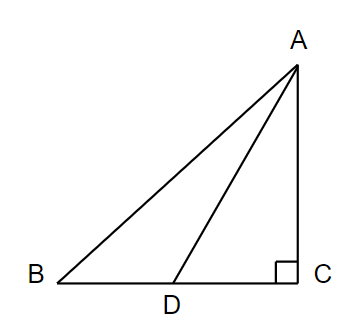QUESTION

# In a right angled triangle $ABC$ in which $\angle C=90$, if $D$ is the midpoint of $BC$, prove that $A{{B}^{2}}=4A{{D}^{2}}-3A{{C}^{2}}$.

Hint: Consider triangle $ADC$ first and use Pythagoras theorem, then take triangle $ABC$ and apply Pythagoras theorem. Now apply the fact that $DC$ is $\dfrac{1}{2}BC$ and substitute the equations to get the answer.

In the question, a right-angle triangle is given named $ABC$, where angle $C=90{}^\circ$. It is also given that $D$ is the midpoint on $BC$ such that it bisects $BC$ into two halves. So, according to the given information, let us draw the figure first.In this figure, angle $C=90{}^\circ$ and $BD=DC$. Let us consider the triangle $ADC$ first. The angle $C$ is $90{}^\circ$ in this, so we can use the Pythagoras theorem. By applying the Pythagoras theorem in triangle $ADC$, we get, $A{{D}^{2}}=A{{C}^{2}}+D{{C}^{2}}$.
We can also write it as,
$A{{C}^{2}}=A{{D}^{2}}-D{{C}^{2}}.........(i)$
Now, we will consider triangle $ABC$. The angle $C$ in this is also $90{}^\circ$, so we can use the Pythagoras theorem. By applying Pythagoras theorem in triangle $ABC$, we get,
$A{{B}^{2}}=A{{C}^{2}}+B{{C}^{2}}.........(ii)$
Substituting the value of $A{{C}^{2}}$ from equation (i) in equation (ii), we get,
$A{{B}^{2}}=A{{D}^{2}}-D{{C}^{2}}+B{{C}^{2}}.........(iii)$
We know that, $BD=DC$ and $DC=\dfrac{1}{2}BC$.
Substituting this in equation (iii), we get,
\begin{align} & A{{B}^{2}}=A{{D}^{2}}-\dfrac{B{{C}^{2}}}{4}+B{{C}^{2}} \\ & \Rightarrow A{{B}^{2}}=A{{D}^{2}}+\dfrac{3}{4}B{{C}^{2}} \\ \end{align}
On cross multiplying, we get,
$4A{{B}^{2}}=4A{{D}^{2}}+3B{{C}^{2}}.........(iv)$
From equation (i), we have,
$A{{C}^{2}}=A{{D}^{2}}-D{{C}^{2}}$
So, by substituting $DC$ with $\dfrac{1}{2}BC$, we get,
$A{{C}^{2}}=A{{D}^{2}}-{{\left( \dfrac{1}{2}BC \right)}^{2}}$
By multiplying by 4 across the equation, we get,
$4A{{C}^{2}}=4A{{D}^{2}}-B{{C}^{2}}$
Which can be written as,
$B{{C}^{2}}=4A{{D}^{2}}-4A{{C}^{2}}.........(v)$
By substituting equation (v) in equation (iv), we get,
$4A{{B}^{2}}=4A{{D}^{2}}+3\left( 4A{{D}^{2}}-4A{{C}^{2}} \right)$
On further simplification, we get,
$4A{{B}^{2}}=4A{{D}^{2}}+12A{{D}^{2}}-12A{{C}^{2}}$
Simplifying again, we get,
$4A{{B}^{2}}=16A{{D}^{2}}-12A{{C}^{2}}$
By dividing the equation throughout by 4, we get,
$A{{B}^{2}}=4A{{D}^{2}}-3A{{C}^{2}}$
Hence it is proved.

Note: The students should be careful while applying the Pythagoras theorem in a triangle. They should use the equations and their forms carefully to get the answer in the least number of steps. we interchange the value of sides in terms of prevoius one to find solution.